Ch 4. Particle Momentum and Impulse Multimedia Engineering Dynamics Impulse & Momentum Consv. Linear Momentum Impact AngularMomentum MassFlow
 Chapter - Particle - 1. General Motion 2. Force & Accel. 3. Energy 4. Momentum - Rigid Body - 5. General Motion 6. Force & Accel. 7. Energy 8. Momentum 9. 3-D Motion 10. Vibrations Appendix Basic Math Units Basic Equations Sections Search eBooks Dynamics Fluids Math Mechanics Statics Thermodynamics Author(s): Kurt Gramoll ©Kurt GramollDYNAMICS - CASE STUDY SOLUTION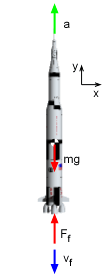Force and Acceleration Diagram of Rocket Use Newton's Second Law to sum all the forces acting on the rocket and equate it to the acceleration times mass,      ΣF = ma      Ff - mg = m (dv/dt) The thrust, Ff, is due to the mass flow of the burning propellant. Using the mass flow equation gives,      Ff = -(dmf /dt) vf = (dmf /dt) vfj = cvfj Notice, all motion and forces are only in the y direction. Combining equations for the y-direction,      cvf - mg = m (dv/dt) Rearrange to get      dv = (cvf /m - g)dt At any time t, the total mass of the rocket and the remaining fuel can be expressed as      m = mo - (dmf /dt) t = mo - ct By substituting, the change in velocity, dv, is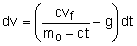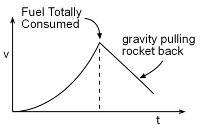Plot of Rocket Velocity Equation Integration will give an expression for the velocity as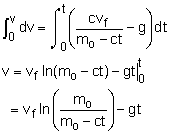The total time required to burn all the fuel is      mf = (dmf /dt)ttot = c ttot      ttot = mf /c Now that the time needed to burn all the fuel is known, it can be substituted back into the velocity equation to give,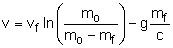where vf = fuel velocity (constant)          mo = initial mass of rocket and fuel          mf = initial mass of fuel          c = fuel burn rate, dmf/dt

Practice Homework and Test problems now available in the 'Eng Dynamics' mobile app
Includes over 400 problems with complete detailed solutions.
Available now at the Google Play Store and Apple App Store.